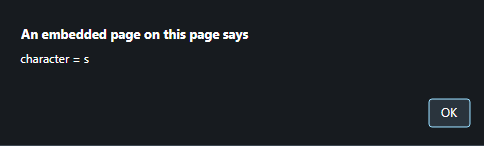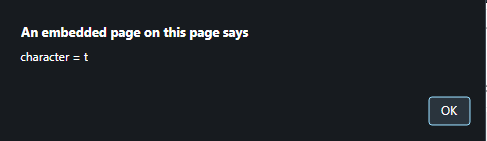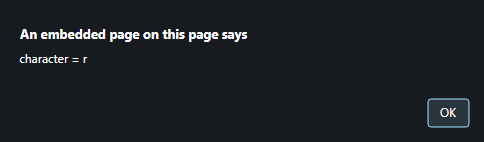# How to process each letter of text using JavaScript ?

Given a string and the task is to process it character by character.

• JavaScript String toUpperCase() Method: This method converts a string to uppercase letters.
Syntax:

```string.toUpperCase()
```

Parameters: This method accepts single parameter str which is required. It specifies the string to be searched.

Return Value: It returns a string denoting the value of a string converted to uppercase.

• JavaScript String charAt() Method: This method returns the character at the passed index in a string. The index of characters strarts from 0.

Syntax:

```string.charAt(index)
```

Parameters: This method accepts single parameter index which is required. It specifies the integer representing the index of the character to return.

Return Value: It returns a string, denoting the character at the passed index, or an empty string if the index number is invalid.

Example 1: This example processes the string letter by letter using for loop and converts each letter to uppercase separately.

 `  ` `<``html``>  ` `    ``<``head``>  ` `        ``<``title``>  ` `            ``How to process each character of text ` `        `` ` `    ``  ` `     `  `    ``<``body` `style` `= ``"text-align:center;"``>  ` `     `  `        ``<``h1` `style` `= ``"color:green;"` `>  ` `            ``GeeksForGeeks  ` `        `` ` `     `  `        ``<``p` `id` `= ``"GFG_UP"` `style` `=  ` `            ``"font-size: 15px; font-weight: bold;"``> ` `        `` ` `         `  `        ``<``button` `onclick` `= ``"gfg_Run()"``>  ` `            ``check ` `        `` ` `         `  `        ``<``p` `id` `= ``"GFG_DOWN"` `style` `=  ` `            ``"color:green; font-size: 20px; font-weight: bold;"``> ` `        `` ` `         `  `        ``<``script``> ` `            ``var el_up = document.getElementById("GFG_UP"); ` `            ``var el_down = document.getElementById("GFG_DOWN"); ` `            ``var str = "This is String"; ` `            ``el_up.innerHTML = "String = '"+str + "'"; ` `         `  `            ``function gfg_Run() { ` `                ``var str_Upper = ""; ` `                ``for (var i = 0; i < ``str.length``; i++) { ` `                    ``str_Upper += str.charAt(i).toUpperCase(); ` `                ``} ` `                ``el_down.innerHTML` `= ``str_Upper``; ` `            ``}          ` `        ``  ` `    ``  ` `                     `

Output:

• Before clicking on the button:• After clicking on the button:Example 2: This example processes the string letter by letter using for in loop and alerts each letter separately.

 `  ` `<``html``>  ` `    ``<``head``>  ` `        ``<``title``>  ` `            ``How to process each letter of text ` `        `` ` `    ``  ` `     `  `    ``<``body` `style` `= ``"text-align:center;"``>  ` `     `  `        ``<``h1` `style` `= ``"color:green;"` `>  ` `            ``GeeksForGeeks  ` `        `` ` `     `  `        ``<``p` `id` `= ``"GFG_UP"` `style` `=  ` `            ``"font-size: 15px; font-weight: bold;"``> ` `        `` ` `         `  `        ``<``button` `onclick` `= ``"gfg_Run()"``>  ` `            ``check ` `        `` ` `         `  `        ``<``script``> ` `            ``var el_up = document.getElementById("GFG_UP"); ` `            ``var str = "str"; ` `            ``el_up.innerHTML = "String = '" + str + "'"; ` `         `  `            ``function gfg_Run() { ` `                ``for (var i in str) { ` `                    ``alert(" character = "+str.charAt(i)); ` `                ``} ` `            ``}          ` `        ``  ` `    ``  ` `                     `

Output:

• Before clicking on the button:• After clicking on the button:My Personal Notes arrow_drop_upCheck out this Author's contributed articles.

If you like GeeksforGeeks and would like to contribute, you can also write an article using contribute.geeksforgeeks.org or mail your article to contribute@geeksforgeeks.org. See your article appearing on the GeeksforGeeks main page and help other Geeks.

Please Improve this article if you find anything incorrect by clicking on the "Improve Article" button below.